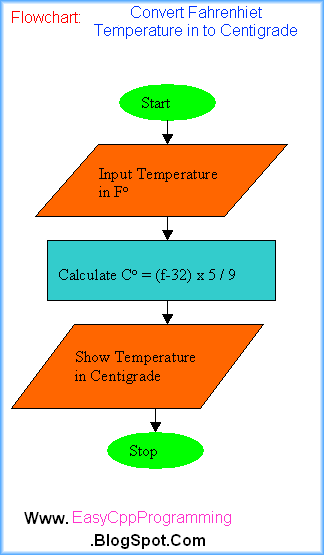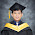# C++ Tutorial With Basic Programs for Beginners

C++ tutorial, C++ programs, C++ code, C++ flowcharts, C++ statements, C++ syntax, C++ examples

## Q: Write a C++ program To Convert Fahrenheit Temperature into Centigrade

Today, we will discuss a Program Temperature Conversion Algorithm Flowchart. This is a Three in One package for new C++ programmers for learning How To Program in C++. Let us see the algorithm first:

## Algorithm: Temperature Conversion

This algorithm inputs a temperature in Fahrenheit and converts into centigrade.

1. Start
2. Input Temperature in Fahrenheit in F variable.
3. Calculate Centigrade C = (F-32) x 5.0 / 9.0
4. Show temperature in centigrade
5. Stop

## Picture of Temperature Conversion C++ Program With Sample Run Output

#### Image: Program Temperature Conversion Algorithm Flowchart## Flowchart of C++ Temperature Conversion Program

#### Image: ( Flow chart )Program Temperature Conversion Algorithm Flowchart## Source code for DevC++ IDE/ C++ Compiler

```#include<iostream>

using namespace std;

int main()

{

float c,f;

cout<< "Enter Temperature in F:";
cin>>f;
c=(f-32)*5.0/9.0;

cout<<"Temperature in centigrade="<<c;
return 0;
}
```

### Output

```Enter Temperature in F:98.6
Temperature in centigrade=37
--------------------------------
Process exited after 16.38 seconds with return value 0
Press any key to continue . . .
```

## C++ Source code Program Temperature Conversion

Sponsored Links

// include iostream.h header file

#include<iostream.h>

// start of main function

int main()

{       // start of main function body
// declare 2 float variables c and f

float c,f;

//show message to user to enter temperature in F
cout<< "Enter Temperature in F:";

//input temperature in F
cin>>f;

//calculate temperature in centi grade
c=(f-32)*5.0/9.0;

//show answer in centigrade
cout<<"Temperature in centigrade="<<c;

// return 0 - successful execution

return 0;
}     // end of main function body

So, beginners in C++ Programming, we have discussed how to design an algorithm, flow chart and write actual program code. Dear learners of C++, this is the end of this C++ tutorial on Program Temperature Conversion Algorithm Flowchart.

### Simple Temperature Conversion program without comments C++ on Easyway C++

//Turbo C++ 3.0 IDE Easyway C++ Programs
#include<iostream.h>
#include<conio.h>
void main()
{

float c,f;

cout<< "Enter Temperature in F:";

cin>>f;

c=(f-32)*5.0/9.0;

cout<<"Temperature in centigrade="<<c;

getche();
}

Further Suggested Readings: After Download Free Turbo C++ Ver 3.0 IDE!

Basic Structure of a C++ Program

C++ Program Development Cycle

How To Write, Compile and Run Your First C++ Program

How To Start Turbo C++ IDE

How To Install Turbo C++ 3.0 - IDE

Features of C++ Programming Language

Brief History of C++

Flowcharts in Computer Programming

The Role of Algorithms in Programming

How To Start Computer Programming

What is a Computer Program

- See more at: http://easycppprogramming.blogspot.com
< /b>

Share:

#### 2 comments:

1.hey may i ask?

2.Great work, on C++ programs , let us C program f into c degree

We Love To Hear From You!